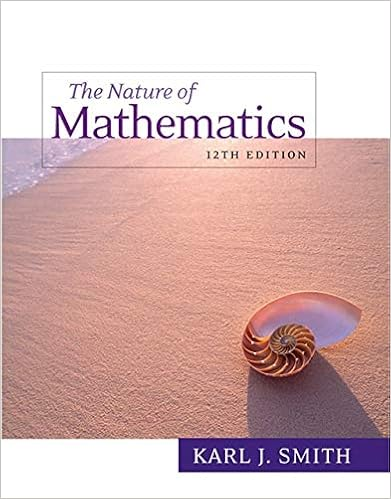# What is the negation of the following statement i

• 14
• 96% (46) 44 out of 46 people found this document helpful

This preview shows page 9 - 10 out of 14 pages.

##### We have textbook solutions for you!
The document you are viewing contains questions related to this textbook.The document you are viewing contains questions related to this textbook.
Chapter 3 / Exercise 24
Nature of Mathematics
SmithExpert Verified
C. If n is divisible by both 2 and 3 then n is not divisible by 6. D. If n is not divisible by both 2 and 3 then n is not divisible by 6. E. If n is divisible by 6 then n is not divisible by both 2 and 3. F. If n is divisible by 6 then n is divisible by both 2 and 3. What is the contrapositive of the following: ”If P is a square then P is a rectangle.” A. If P is a square then P is not a rectangle. B. If P is a rectangle then P is a square. C. If P is a rectangle then P is not a square. D. If P is not a rectangle then P is not a square. E. If P is not a square then P is not a rectangle. F. If P is a square then P is a rectangle.Expert Verified
•••